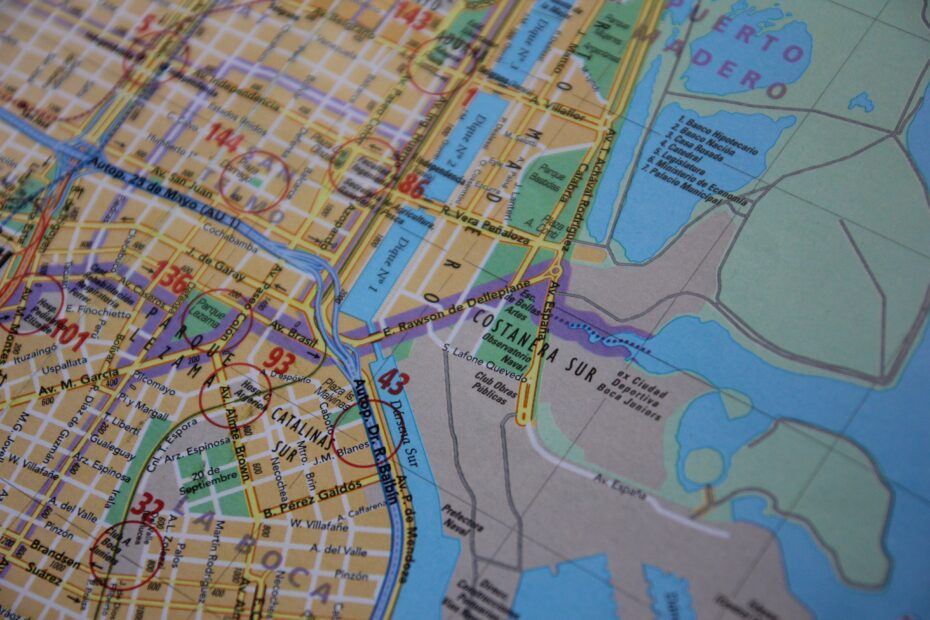# Easy way to detect isolated and overlapping geo polygons in PythonThere are many scenarios in which you have to detect any type of isolated polygons and overlapping polygons. But you also need to make it faster and 100 percent accurate when you pass the large numbers of polygons for processing. In this article, you’ll see exactly how to detect isolated and overlapping geo polygons in Python.

## Why do you need to detect isolated and overlapping geo polygons in python?

There are many cases in which you need to do this. But in geo-spatial analysis, this is highly needed. Suppose, you have created a circle out of a given radius and coordinates, and on that, you want to apply your algorithm only on isolated circles or only on overlapping circles without affecting each other.

Also becomes helpful when you are doing geometry operations(i.e. detecting whether the triangle is intercepting with a square or not) in Python.

## Why not use the Shapely Python library?

Shapely library is a Python package for performing geometry-related operations on top of polygons. It uses functions from the GEOS library to do this.

It has many functions to do geometry-related operations. Like creating a union, checking the equality of any geometry, etc.

In that, there are two functions that could help to detect isolated and overlapping polygons. Which are shapely.intersects and shapely.touches.

Although Shapely provides these functions, it fails miserably in terms of speed when it encounters a large number of polygons as an input.

Therefore you can use these Shapely’s functions if you are not dealing with big numbers of polygons. Otherwise, it will take too much time to process.

## Solution for detecting non-overlapping and overlapping geo polygons

First, you have to generate polygons. Let’s assume that you have generated polygons. In my case, I have generated 2000 polygons out of coordinates and radii and stored them in the `circles` column of the Dataframe. (If you don’t know how to generate circles out of coordinates and radii, I’d highly suggest checking this article)

As our data is related to geospatial analysis, Let’s see how they are looking on Kepler.gl. (Kepler.gl is a powerful open-source geospatial analysis tool for large-scale data sets.)

Now, let’s do the main part of detecting single polygons and polygons which are overlapping with each other.

```import geopandas as gpd
from rtree import index

def extraction_single_points(df):
"""
extraction of single circles from all circles
:param df: dataframe of latitude,longitude,radius,location_ID and circles
"""
polygons = df.circles.values

polygons = gpd.GeoDataFrame(geometry=polygons)
idx = index.Index()
for i, poly in enumerate(polygons.geometry):
idx.insert(i, poly.bounds)

# Find the non-intersecting polygons using the R-tree index
non_intersections = []
for i, poly in enumerate(polygons.geometry):
# Compute the bounding box of the polygon
bbox = box(*poly.bounds)
# Query the index to get the candidate neighbors
neighbors = list(idx.intersection(bbox.bounds))
# Check if any of the candidate neighbors actually intersect with the circle
if not any(polygons.iloc[n].geometry.intersects(poly) for n in neighbors if n != i):
non_intersections.append(polygons.iloc[i])

# Convert the non-intersecting polygons to a GeoDataFrame
non_intersections = gpd.GeoDataFrame(non_intersections)

#returning single point and multipoint
return df[df['circles'].isin(non_intersections.geometry.values)].circles.values,df[~df['circles'].isin(non_intersections.geometry.values)].circles.values
```

Here I have used Geopandas and rtree libraries. These libraries help to do geospatial-related operations very fast (Even faster than Shapely).

The above function will create a GeoDataFrame out of the normal DataFrame. Then it will create an empty index with the `Index()` function of the tree library.

After that, It will query the generated index list of all the polygons and will check the intersection with other polygons. For checking the intersection it will create a boundary box of every polygon with `bbox = box(*poly.bounds)` code. (That’s very memory efficient than checking over the whole polygon).

Then it’ll get the list of its neighbors by using Boudary’s coordinates. And lastly, it will check the intersection with their neighbors. Lastly gives the list of isolated points and overlapping points.

In sample Dataframe it detected three polygons. Let’s see them on Kepler.

As you can see it perfectly detected all the isolated circles. This process is also very fast compared to Shapely’s built-in functions. So you don’t have to worry about timing and memory-related issues at all.